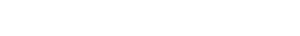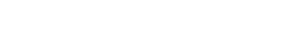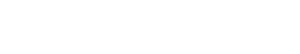3.1.5 Specific Latent Heat

Specific Latent Heat

• During a phase change (i.e. a change of state) thermal energy is transferred to a substance or removed from it, while the temperature of the substance does not change
• In this case, the thermal energy is calculated as follows:

E = mL

• Where:
• m = mass of the substance in kilograms (kg)
• L = specific latent heat of the substance in J kg–1
• The specific latent heat of a substance is defined as:

The amount of energy required to change the state of 1 kg of a substance without changing its temperature

• This definition can be explained when the above equation is rearranged for L:• This means that the higher the specific latent heat of a substance, the greater the energy needed to change its state
• Note that the specific latent heat is measured in J kg–1
• The amount of energy required to melt (or solidify) a substance is not the same as the amount of energy required to evaporate (or condense) the same substance
• Hence, there are two types of specific heat:
• Specific latent heat of fusion, Lf
• Specific latent heat of vaporisation, Lv
• Specific latent heat of fusion is defined as:

The amount of energy needed to melt or solidify a material at its melting point

• This applies to the following phase changes:
• Solid to liquid
• Liquid to solid
• Specific latent heat of vaporisation is defined as:

The amount of energy needed to evaporate or condense a material at its boiling point

• This applies to the following phase changes:
• Liquid to gas
• Gas to liquid
• For the same substance, the value of the specific latent heat of vaporisation is always much higher than the value of the specific latent heat of fusion
• In other words, Lv > Lf
• This is because much more energy is needed to evaporate (or condense) a substance than it is needed to melt it (or solidify it)Worked Example

Determine the energy needed to melt 200 g of ice at 0°C.

• The specific latent heat of fusion of water is 3.3 × 105 J kg–1
• The specific latent heat of vaporisation of water is 2.3 × 106 J kg–1

Step 1: Determine whether to use latent heat of fusion or vaporisation

• We need to use the specific latent heat of fusion because the phase change occurring is from solid to liquid

Step 2: List the known quantities

• Mass of the ice, m = 200 g = 0.2 kg
• Specific latent heat of fusion of water, Lf = 3.3 × 105 J kg–1

Step 3: Write down the equation for the thermal energy

E = mLf

Step 4: Substitute numbers into the equation

E = 0.2 kg × (3.3 × 105) J kg–1

= 6.6 × 104 J = 66 kJ

Worked Example

Energy is supplied to a heater at a rate of 2500 W.

Determine the time taken to boil 0.50 kg of water at 100°C. Ignore energy losses.

• The specific latent heat of fusion of water is 3.3 × 105 J kg–1
• The specific latent heat of vaporisation of water is 2.3 × 106 J kg–1

Step 1: Determine whether to use latent heat of fusion or vaporisation

• We need to use the specific latent heat of vaporisation because the phase change occurring is from liquid to gas

Step 2: Write down the known quantities

• Power, P = 2500 W
• Mass, m = 0.50 kg
• Specific latent heat of vaporisation of water, Lv = 2.3 × 106 J kg–1

Step 3: Recall the equation linking power P, energy E and time t

E = Pt

Step 4: Write down the equation for the thermal energy E

• The energy E in the previous equation is the thermal energy transferred by the heater to the water

E = mLf

Step 5: Equate the two expressions for energy

Pt = mLf

Step 6: Solve for the time tt = 460 s

CloseClose